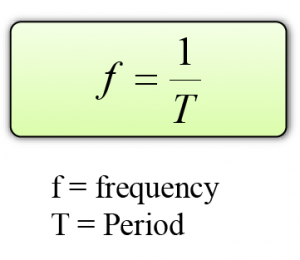# Oscillation

1. Waves are formed by a series of oscillation.
2. In order to understand waves, we must understand oscillation.

### Technical Terms Related to Oscillation

1. An equilibrium position is a point where an oscillating object experiences zero resultant forces.
2. A complete oscillation occurs when the vibrating object:
1. moves to and fro from its original position and
2. moves in the same direction as its original motion.3. Amplitude is the maximum amplitude of an object from its equilibrium position. The SI unit for amplitude is meter, m.4. The greater the amplitude, the greater the mechanical energy possessed by the oscillating system.
5. Period is defined as the time required for one complete oscillation or vibration.
6. Frequency, f is the number of oscillation that takes place in one second. The SI unit for frequency is Herz (Hz).
7. Frequency can be related to the period by the following equationExample:
Given that a pendulum makes 20 oscillations in 25s. Find the frequency of the pendulum.

### 2 thoughts on “Oscillation”

1.nice~thx you

2.what is the meaning T f= 1 1.25 =0.8Hz? i cant understand T.T